Change Your Codes Behavior By Using Bitwise Operators by@silver.io

# Change Your Codes Behavior By Using Bitwise OperatorsLet’s dissect a weird bit flag program that took me a second to understand. in doing so, we’ll hopefully gain a more robust understanding of how bit masks and bitwise OR logic can manipulate values effectively.

Specifically, the program we’ll look at prints the binary representation of different access mode flags that are used in the open() function (included in the <

``fcntl.h``
> library). The access mode flags function to specify the permissions of the file that’s getting opened or created. The flags have “values that correspond to single bits”, (Hacking: The Art of Exploitation, Jon Erickson) and consequently, the flags can be added together (using the OR operator) to create new behavior. Let’s take a look at the following program: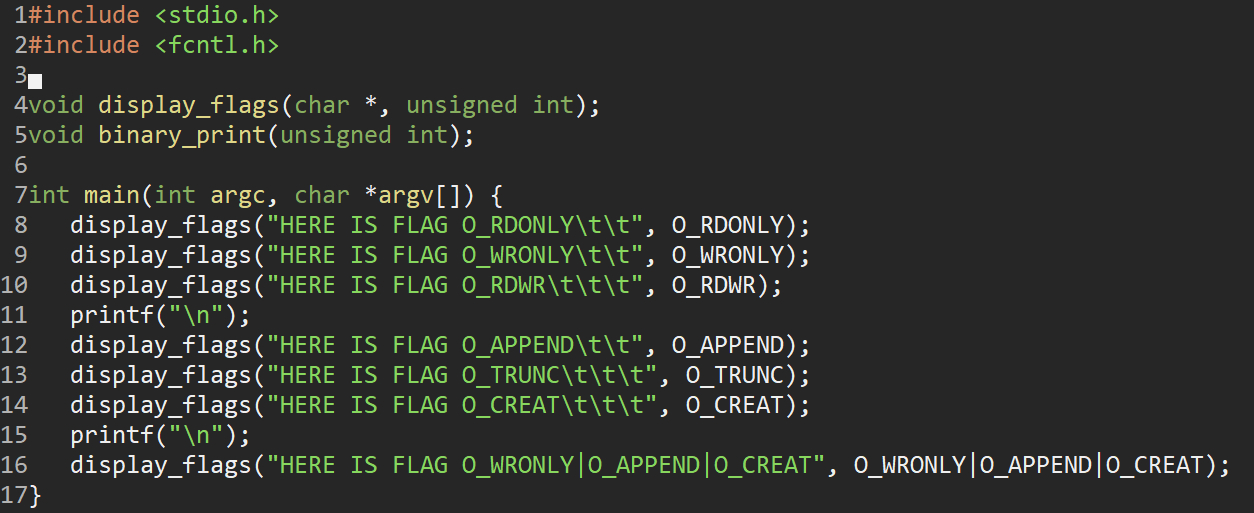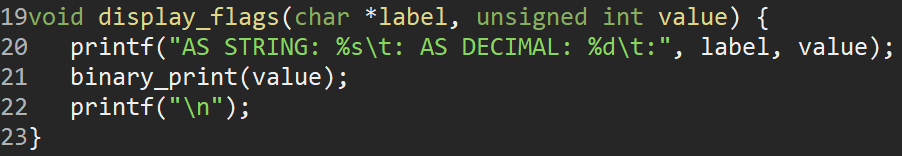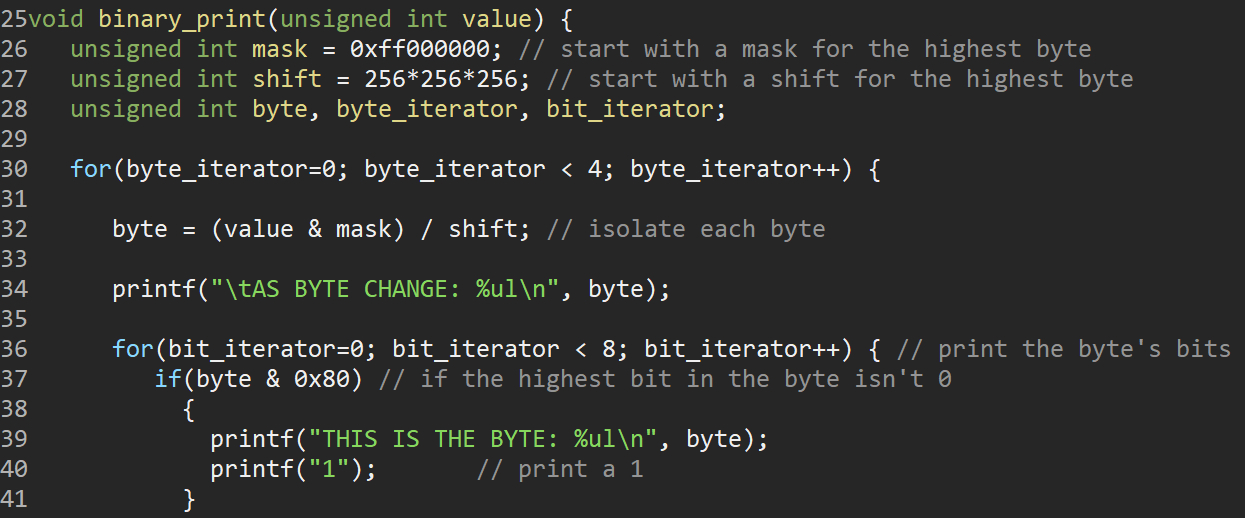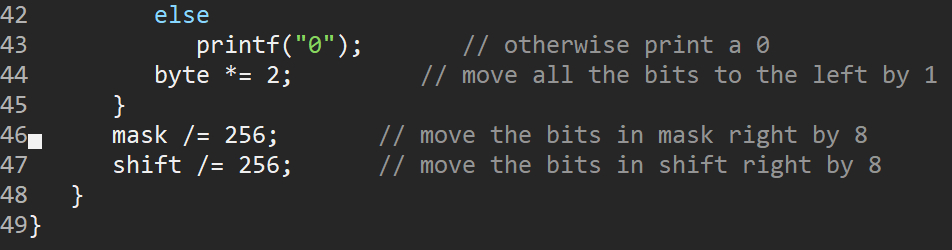The first time I saw this program I didn’t understand what it was doing — so let’s walk through it. First, the main() function sends the value of the access mode flags to the display_flags() function.

The display_flags function prints the string and decimal value of the access mode flag. Display_flags() then sends the decimal value of the access mode flag to binary_print().

Binary_print() has an inner and outer loop structure. We use a bit mask (the “mask” variable) with a value of 4278190080 (this number requires four bytes). In binary, this mask looks like eight ones followed by twenty four zeros:

``11111111000000000000000000000000``

We then have a variable “shift” with a value of 16777216 which looks like a one followed by twenty-four zeros in binary:

``1000000000000000000000000``

The outer loop runs four times — and is in charge of isolating each byte. The first line of code inside the outer loop:

``byte = (value & mask) /shift;``

Does a bitwise AND operation with the value of the access mode flag and the value of the mask. The product of the resulting operation is than divided by shift, so that only the eight leftmost bits are saved. Here’s an example:

``````value = 10000000000
shift = 100000000
value
______________ = 10000000000 / shift = 00000100``````

In this case the byte variable will hold a value of 4.

Once we’ve isolated each byte, we move to the inner loop. The inner loop iterates through each bit in the byte and checks whether the msb (most significant bit (leftmost bit)) is set. If it is, we print a “1”. If it’s not, we print a “0". After printing the bit we shift the byte leftward (getting a new msb) by multiplying the byte by two:

``````byte *= 2
Ex. Four becomes eight:
A = 00000100
A *= 2
A = 00001000``````

Following the inner loop, we divide both the mask and the shift variables by 8 in order to move the bits to the right by eight bits. We do this to check if lower order bits in our value are set. We repeat this process: isolating the next byte, checking if the msb is set, and shifting the bits to the left so we can check the next msb for four bytes.

At the end of this process, we will have the full binary representation of the access mode flag. While this in itself is not very useful, it might be helpful in further low-level programming endeavors to have a function that can quickly produce a binary representation of whatever you’re looking at. If nothing else, it’s good to have a solid understanding of how bits are moving in your program as it might allow you to think about ways you can optimize the time and space complexity of your code.

Before the end, I’d like to demonstrate how

``OR``
bitwise operators can be used as an addition operator. The
``O_WRONLY``
flag has a value of 1, the
``O_APPEND``
flag has a value of 1024, and the
``O_CREAT``
flag has a value of 64. When we call display_flags() and print the decimal representation of
``O_WRONLY``
|
``O_APPEND``
|
``O_CREAT``
, we get 1089 (the same as adding them together). This will work always, as long as all the bits in each of the numbers are unique.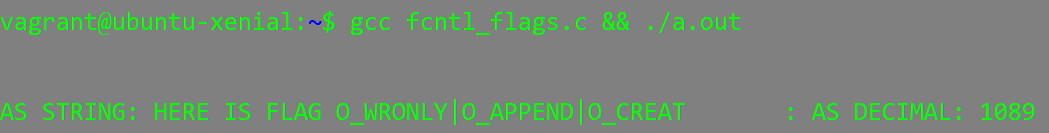Join Hacker Noon